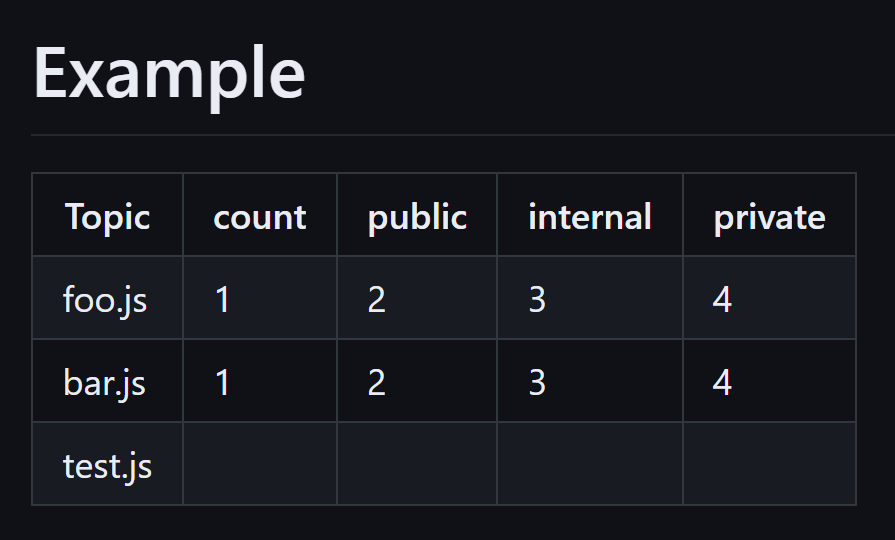Date posted: 08 Jun 2023, 3 minutes to read

# Writing to the \$GITHUB_STEP_SUMMARY with the core npm package

Every time I need to write to the GITHUB_STEP_SUMMARY in GitHub Actions from the actions/github-script action (or from Typescript), I need to search for the blogpost that announced it’s existence. So I’m writing this blogpost to make it easier for myself to find it a lot easier, including some working examples.##### Photo by Markus Winkler on Unsplash

The code for the summaries lives in the actions/core package on npm, but figuring out how to use it can be a bit hard. The only example I’ve seen is in the blogpost I mentioned.

``````  import * as core from '@actions/core'

await core.summary
['foo.js', 'Pass ✅'],
['bar.js', 'Fail ❌'],
['test.js', 'Pass ✅']
])
.write()
``````

This does a lot of things at the same time, but we get the general idea that you can:

• add links And at the end you need to write the summary itself, which will be added to the file in the GITHUB_STEP_SUMMARY environment variable.

## Working with the table output

There are no methods to break the table into chunks, like:

The only method there is, is adding the table in one go, with each row as an array of objects, and some configuration in the first row as that will define if the cell is a header or not. So assuming you have an array of results that you want to show, you can convert that array with properties into an array of rows, with each property value being an item in the row array.

The interesting thing I ran into, is that the row cells must be a string. Sending in integers for example does not work. Take the following example:

``````await core.summary
['foo.js' , "1", "2"],
['bar.js' , '3', '4'],
['test.js', 100, 200]
])
.write()
``````

In this example, all rows will be added to the summary, and as long as the content is a valid string, it will be shown in the table as well. In this example, the values in the last row are integers, and they will be not visible in the table.A full example of creating the header array with hardcoded cells, and then adding the rows from an array of objects can be seen below. Here I have an array stored as output in a previous step, so I read that file and map it (as string values!) to an array containing the rows. The next step is to join the two arrays (header + summary) and pass that to the addTable method.

``````- name: Show information in the GITHUB_STEP_SUMMARY
uses: actions/github-script@v6
env:
summaryFile: \$
with:
script: see below in other markup for better readability
``````
``````        const fs = require('fs')

// make the heading array for the core.summary method

// convert the summary array into an array that can be passed into the core.summary method
const summaryArray = JSON.parse(summary).map(t => [t.name, t.count.toString(), t.public.toString(), t.internal.toString(), t.private.toString()])

// join the two arrays